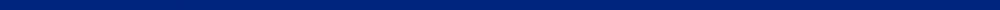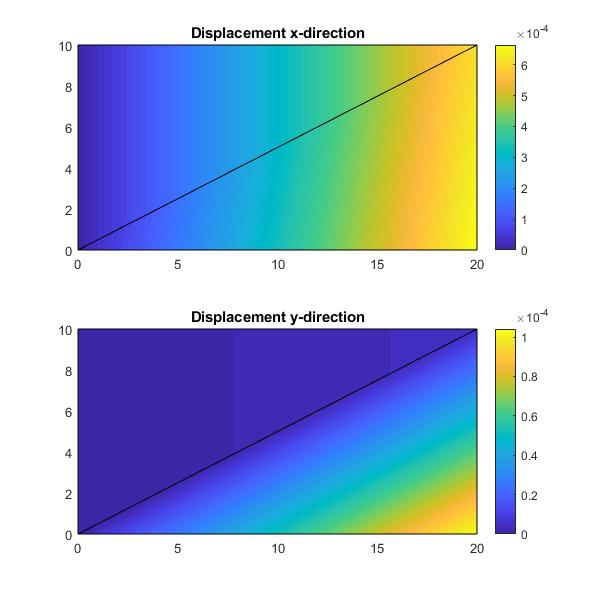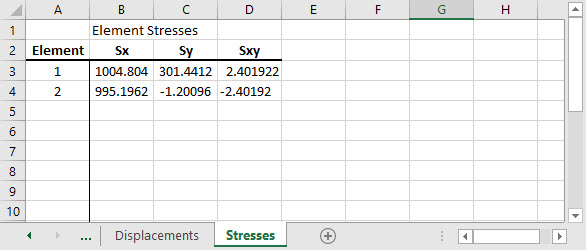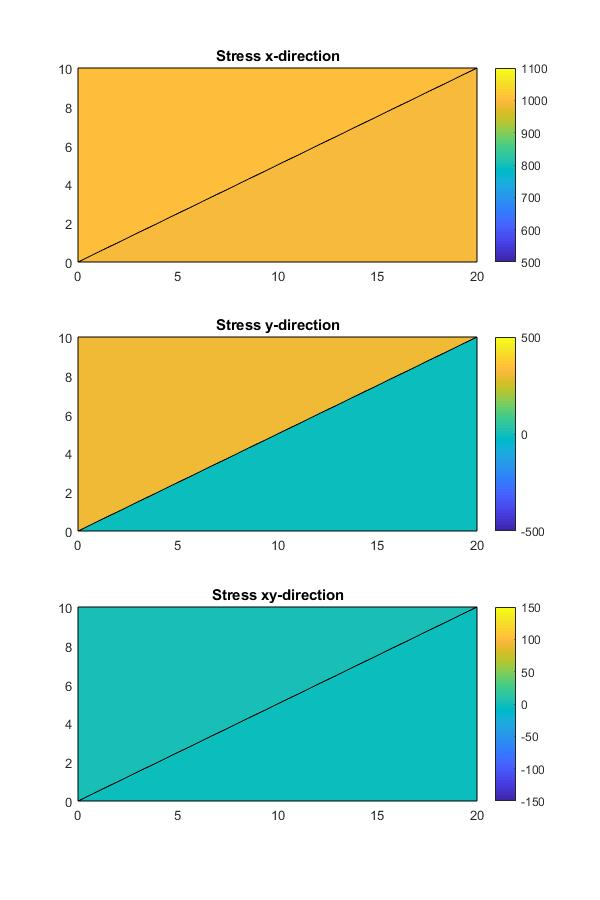Camp's Matlab codesTo download these files, right-click the link and Save As...  Plane stress - plane strain using constant strain triangular elements (CST), 6 node triangular elements (LST), 4 node quadrilateral elements (Q4), and 8-node quadrilateral elements (Q8). Also, axisymmetric plane stress using constant strain triangles (CST_axisym). All of this scripts use an input files structure contained in a Excel spreadsheet with 8 work sheets defining the parameters of the problems: Plane stress-strain: 1 for plane stress or 2 for plane strain Coordinates: x and y coordinates for each node Connectivity: nodal values for  i, j, and m for each element Forces: x and y values for nodal forces Boundary: x and y displacement boundary condition (1 for fixed and 0 for free) Thickness: values for thickness of each element Elasticity: value for modulus of elasticity E for each element Poisson: value for v for each elementDisplacements and stresses are printed into the input Excel spreadsheet. Below are the results for the CST solution using 2 element for the lane stress problem given on page 364 of Logan's "First Course in the Finite Element Method" 6th edition.This web site was originally developed by Charles Camp for CIVL 7117. This site is Maintained by the Department of Civil Engineering at the University of Memphis. Your comments and questions are welcomed.